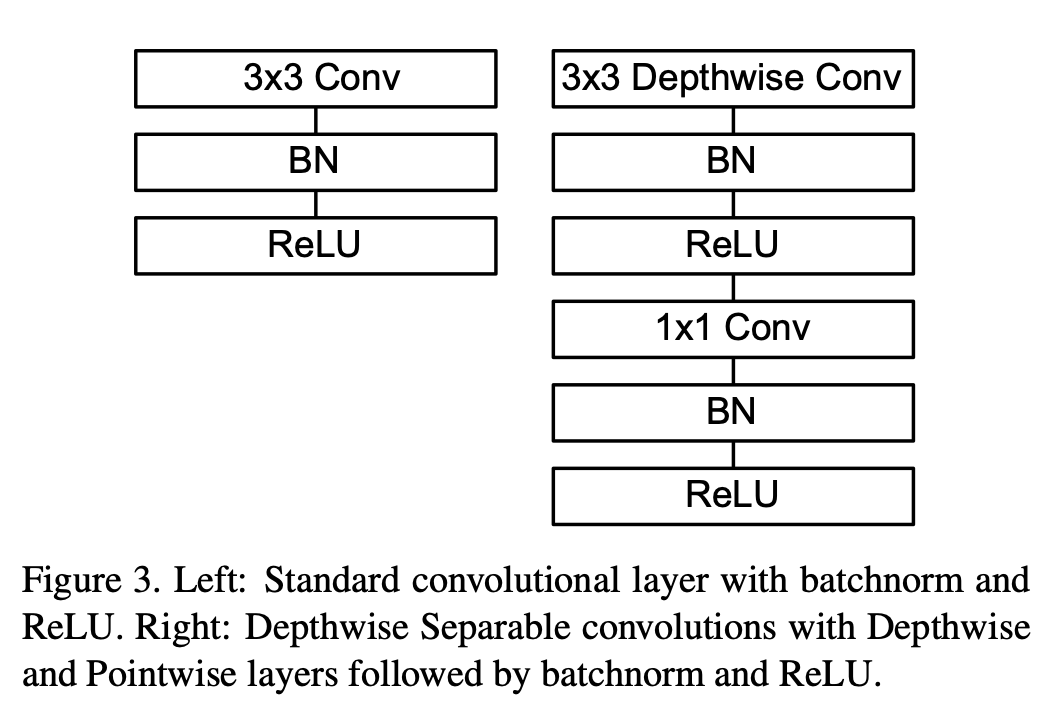# 可分离卷积（Separable Convolution）¶

## 二、空间可分离卷积¶

$\begin{split} \left[\begin{array}{ccc} 3 & 6 & 9 \\ 4 & 8 & 12 \\ 5 & 10 & 15 \end{array}\right] = \left[\begin{array}{ccc} 3 \\ 4 \\ 5 \end{array}\right] \times \left[\begin{array}{ccc} 1 \quad 2 \quad 3 \end{array}\right] \end{split}$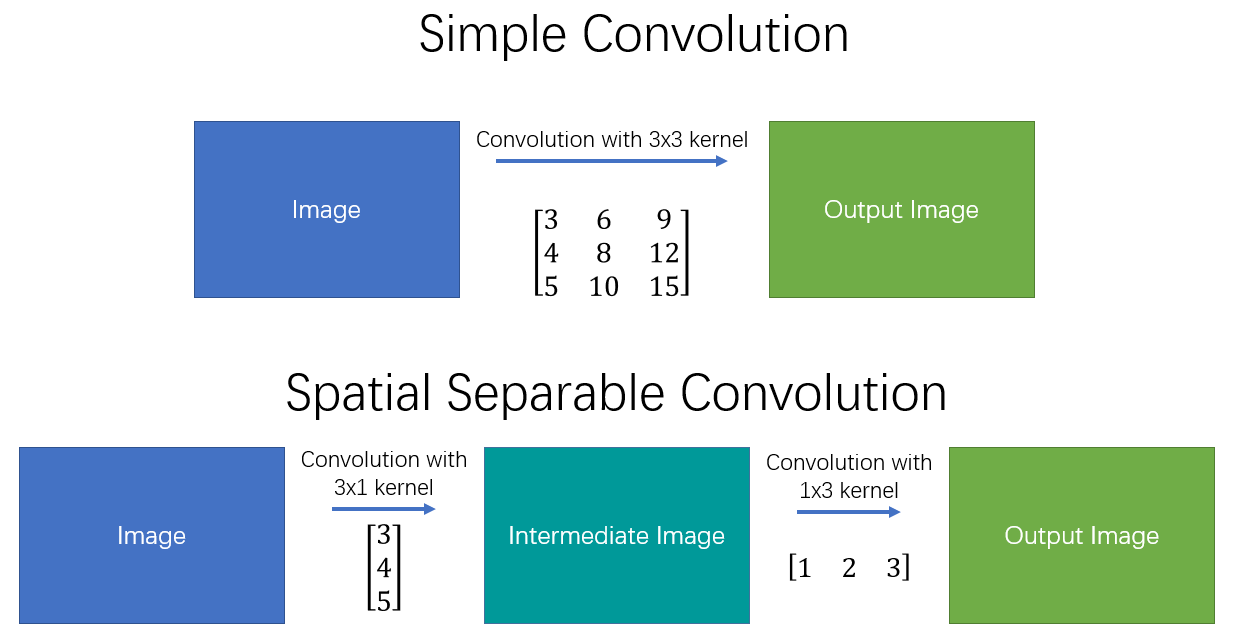### 应用示例¶

$\begin{split} \left[\begin{array}{ccc} -1 & 0 & 1 \\ -2 & 0 & 2 \\ -1 & 0 & 1 \end{array}\right] = \left[\begin{array}{ccc} 1 \\ 2 \\ 1 \end{array}\right] \times \left[\begin{array}{ccc} -1 \quad 0 \quad 1 \end{array}\right] \end{split}$

## 三、深度可分离卷积¶

### 标准卷积¶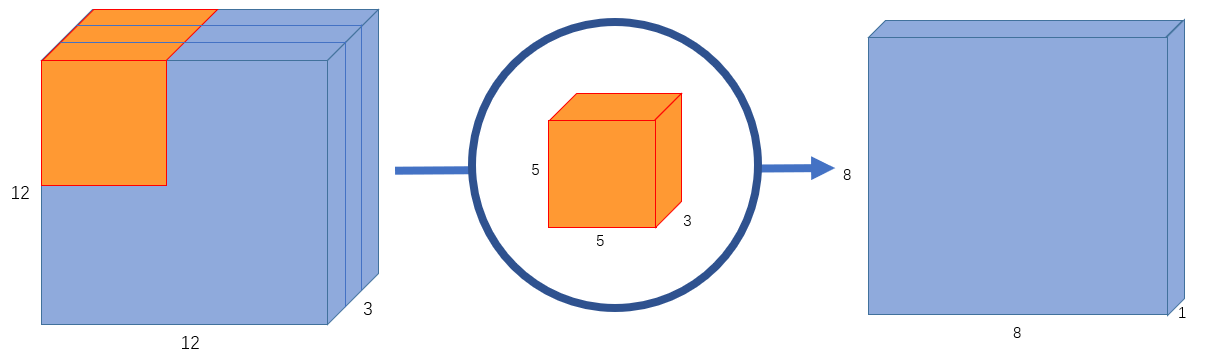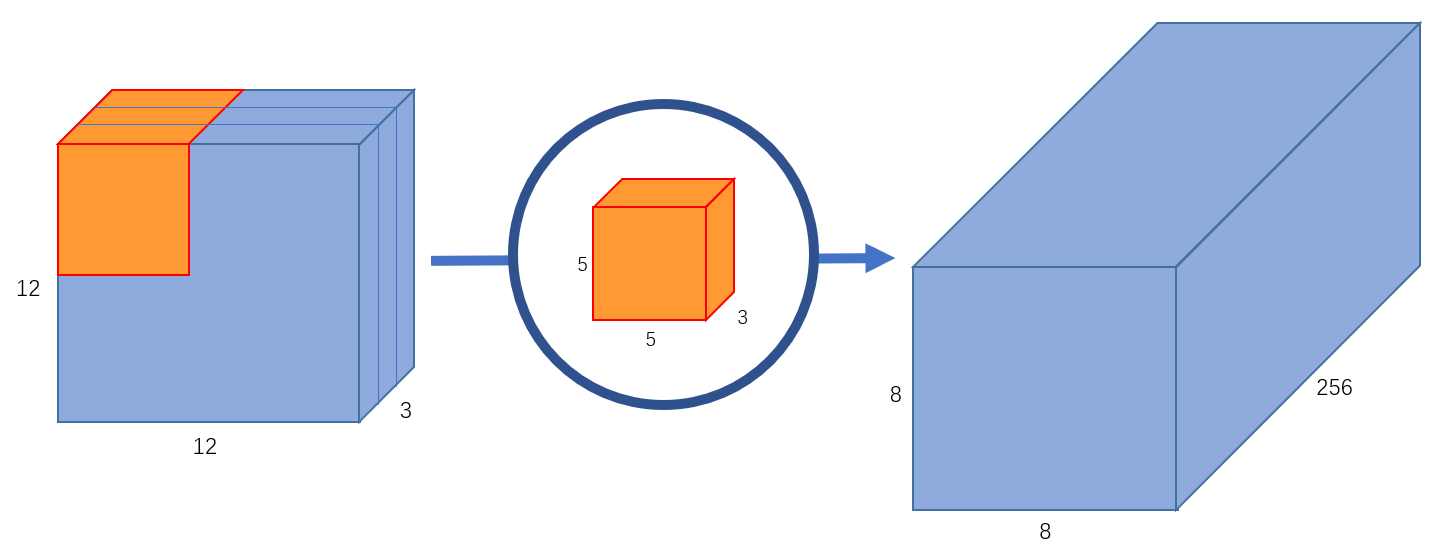### 深度卷积运算¶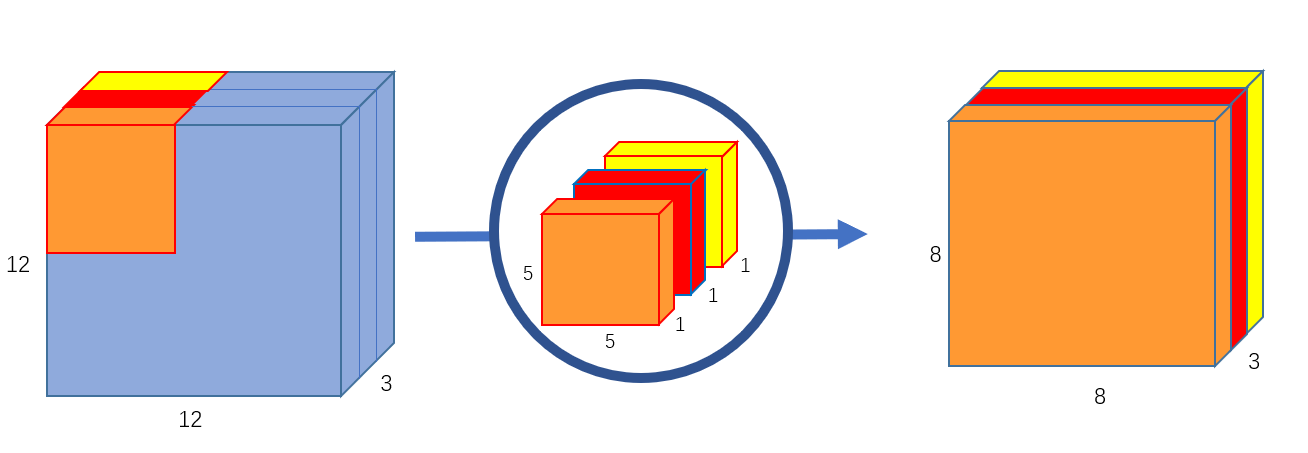### 逐点卷积运算¶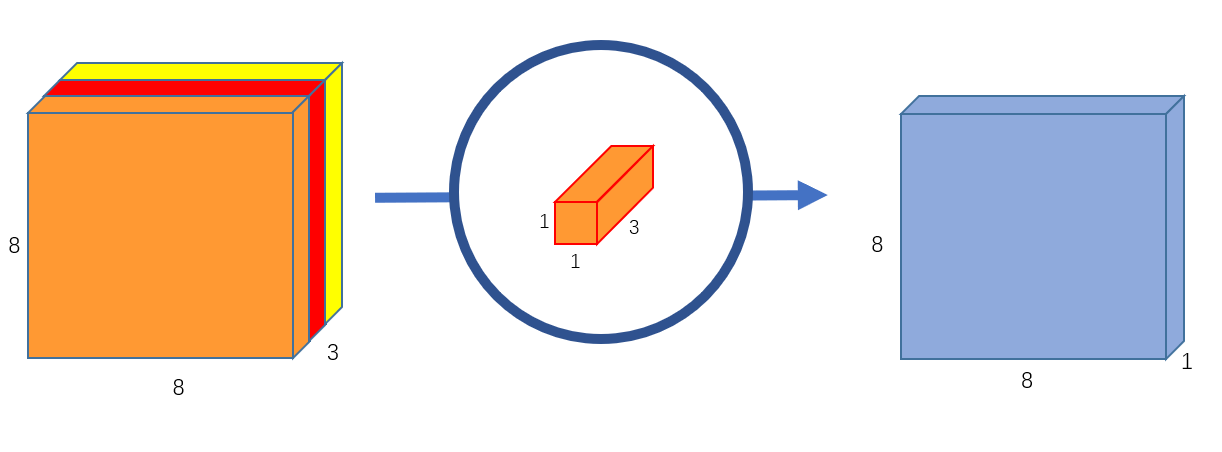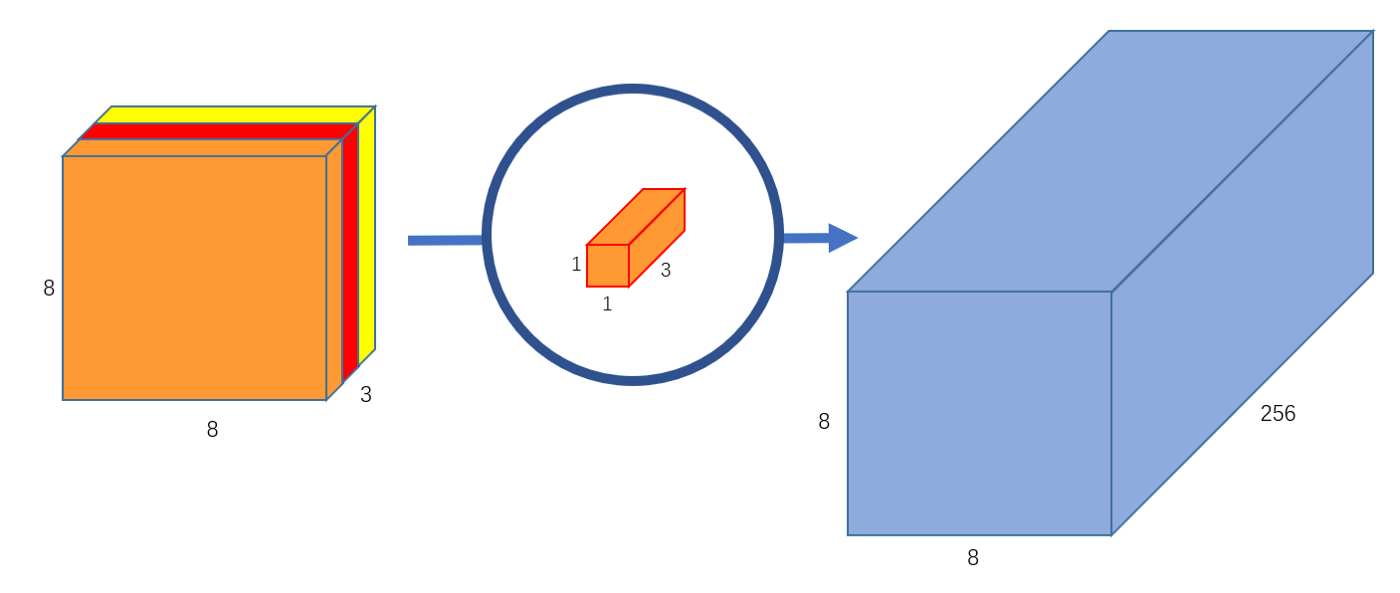### 深度可分离卷积的意义¶

$256 \times 3 \times 5 \times 5 \times 8 \times 8=1228800$

$3 \times 5 \times 5 \times 8 \times 8=4800$

$256 \times 1 \times 1 \times 3 \times 8 \times 8=49152$

### 应用示例¶

MobileNetv1中使用的深度可分离卷积如 图7 右侧所示。相较于左侧的标准卷积，其进行了拆分，同时使用了BN层以及RELU激活函数穿插在深度卷积运算和逐点卷积运算中。